So far we generated noise that goes on forever. But in some cases we want noise that repeats itself after a certain distance though, mainly when we’re baking noise into a texture. In this tutorial I’ll show you how to make noise repeat and how to use uv coordinates instead of worldspace positions for noise generation.

I use the layered perlin noise and voronoi noise to show the theory behind tiling noise, but it’s possible to use those patterns with many different types of noise and other shaders as well. That being said this tutorial is part of the noise series so if you have troubles with anything and haven’t read earlier noise tutorials yet, I recommend you read them first.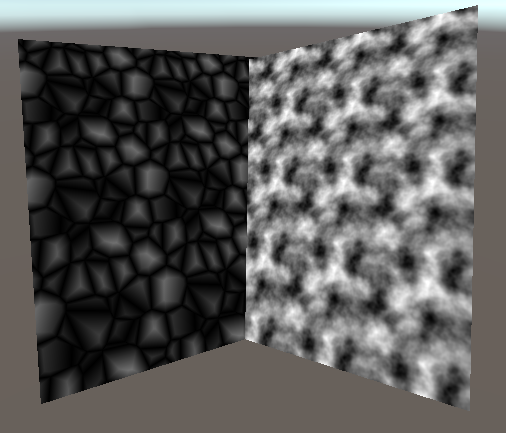## Tileable noise

We’ll use the layered 2d perlin noise as the first shader to modify to be tilable. We expand the perlin function to take another parameter called the period which is how often the noise tiles, counted in cells. Previously we calculated the cell positions directly before we passed them to the random function to calculate the direction of the cell, but to better be able to repeat the noise we’ll calculate the maximum and minimum of the cells before passing it and then choosing the correct parameters out of them. We still get the component minimum of the cells by using floor and get the maximum via ceil. Then we use the x or y component of the minimum vector if we previously used floor and use the x or y component of the maximum vector where we previously used ceil.

Then we make the cell positions wrap according to our new period variable. We do that by taking the modulo of the cell variables. The problem with the modulo implementation is that it returns the remainder. The difference between the mathematical remainder and modulo is that the modulo of will always be positive while the remainder of a negative number is negative. for example the modulo between `-5` and `3` would be `1` because if we multiply `3` by `-2` we get `-6` and the modulo is the difference, `1`. In the base of a implementation using the remainder, the result would be `-2` instead because it assumes that it’s allowed to to just use `-1` as a multiplier and then in that case the difference to our divident is `-1`. The point of this small journey into mathematics is that we have the remainder, but we want the modulo. The fix for that is to first, get the remainder, then add the divisor again and take the modulo a second time. We know that after the first remainder the value isn’t lower than our divisor times -1, so by adding the divisor again, we know the value is positive. And after ensuring we have a positive value we can apply the remainder a second time because we know it behaves exactly the same way as the modulo if we have positive values. We’ll add the modulo as a extra function to our shader. The input and output is a `float2` so we can make the x and y coodinate wrap at the same time.

``````float2 modulo(float2 divident, float2 divisor){
float2 positiveDivident = divident % divisor + divisor;
return positiveDivident % divisor;
}
``````

Now that we have this sorted out, we can use this custom modulo function to make make the cells wrap according to the period. The result will be that if we pass `(4, 4)` as a period, the noise will repeat itself every 4 cells, so in the X direction it’ll go `(0, 0), (1, 0), (2, 0), (3, 0), (0, 0), (1, 0), (2, 0), etc...` and similarly in the y direction `(0, 0), (0, 1), (0, 2), (0, 3), (0, 0), (0, 1), (0, 2), etc...`.

``````float perlinNoise(float2 value, float2 period){
float2 cellsMimimum = floor(value);
float2 cellsMaximum = ceil(value);

cellsMimimum = modulo(cellsMimimum, period);
cellsMaximum = modulo(cellsMaximum, period);

//generate random directions
float2 lowerLeftDirection = rand2dTo2d(float2(cellsMimimum.x, cellsMimimum.y)) * 2 - 1;
float2 lowerRightDirection = rand2dTo2d(float2(cellsMaximum.x, cellsMimimum.y)) * 2 - 1;
float2 upperLeftDirection = rand2dTo2d(float2(cellsMimimum.x, cellsMaximum.y)) * 2 - 1;
float2 upperRightDirection = rand2dTo2d(float2(cellsMaximum.x, cellsMaximum.y)) * 2 - 1;

//rest of the function unchanged
``````
``````void surf (Input i, inout SurfaceOutputStandard o) {
float2 value = i.worldPos.xz / _CellSize;
//get noise and adjust it to be ~0-1 range
float noise = perlinNoise(value, float2(4, 4)) + 0.5;

o.Albedo = noise;
}
``````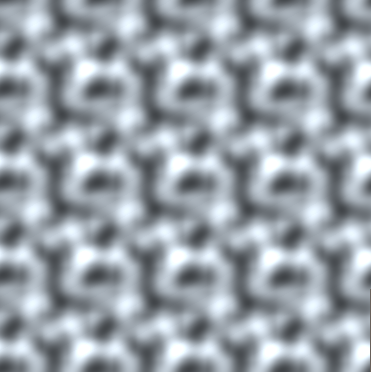## Layered tileable noise

This is all we need for simple tiling noise, but it doesn’t work well for layered noise out of the box. If we double the frequency of the noise to 8 cells in the same area where there were 4 previously, but still repeat the noise every 4 cells, we have a unnessecary amount of repetition and it’ll look weird. To counteract that we have to multiply the period by the frequency in our octaves. Double the amount of cells per space means we can repeat half as frequently.

``````float sampleLayeredNoise(float2 value){
float noise = 0;
float frequency = 1;
float factor = 1;

[unroll]
for(int i=0; i<OCTAVES; i++){
noise = noise + perlinNoise(value * frequency + i * 0.72354, float2(4, 4) * frequency) * factor;
factor *= _Persistance;
frequency *= _Roughness;
}

return noise;
}
``````
``````void surf (Input i, inout SurfaceOutputStandard o) {
float2 value = i.worldPos.xz / _CellSize;
//get noise and adjust it to be ~0-1 range
float noise = sampleLayeredNoise(value) + 0.5;

o.Albedo = noise;
}
``````

Finally we’re going to expose the variable for adjusting the period of the noise to the inspector. The period property is of the type vector. Sadly unity doesn’t allow us to expose vectors other than 4-dimensional ones, but if we define the variable in the hlsl part as a 2d vector it’ll simply ignore the last two components of the property.

Another thing I’d like to change is that currently the roughness is a completely free slider, but for values with a fractional part the tiling breaks. That’s because you can’t cleanly wrap after 3.5 cells for example. To simply forbid ourselves from entering values with fractional parts, we can simply precede the property with the `[IntRange]` attribute.

``````Properties {
_CellSize ("Cell Size", Range(0, 2)) = 2
_Period ("Repeat every X cells", Vector) = (4, 4, 0, 0)
[IntRange]_Roughness ("Roughness", Range(1, 8)) = 3
_Persistance ("Persistance", Range(0, 1)) = 0.4
}
``````
``````//global shader variables
float2 _Period;
``````
``````//sampleLayeredNoise function
noise = noise + perlinNoise(value * frequency + i * 0.72354, _Period * frequency) * factor;
``````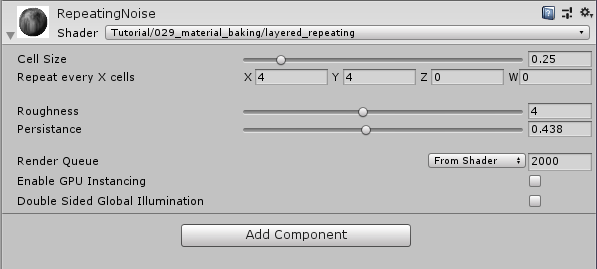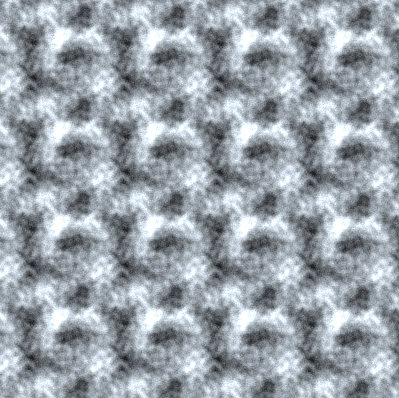## Noise in unlit UV space

So far we always used worldspace coordinates as base values for our noise, specifically to not have to deal with weird scaling or uv mapping on objects. Plus we used surface shaders for super easy access to worldspace coordinates and fancy lighting. But sometimes we want the noise to be in UV space and not use fancy lighting (for example to bake the noise into a texture or to use the cheaper 2d noise on a 3d object). This is how to convert our surface shader into a simpler shader that shows the noise in uv space and doesn’t calculate lighting. (To have the noise in UV space and still have a surface shader for fancy lighting it’s best to add a custom vertex function to the surface shader and pass the uv coordinates into your input struct from there.)

For the change to a non-surface shader, we add a shader pass just around the `CGPROGRAM` hlsl part, we explicitely import UnityCG library file, then we also define the vertex and fragment functions, add the appdata and vertex to fragment structs and lastly we change the surface function to a fragment function and add the vertex function. I copied most of those changes from the code of the tutorial about textures, so if you have any trouble understanding the changes I recommend you reread that.

While doing those changes we can also pass the uv coordinates to the fragment shader and replace the world coordinates with them to have noise in UV space.

``````SubShader {
Tags{ "RenderType"="Opaque" "Queue"="Geometry"}

Pass{
CGPROGRAM

//include useful shader functions
#include "UnityCG.cginc"

//define vertex and fragment shader
#pragma vertex vert
#pragma fragment frag

#pragma target 3.0

#include "Random.cginc"

#define OCTAVES 4

float _CellSize;
float _Roughness;
float _Persistance;
float2 _Period;

//the object data that's put into the vertex shader
struct appdata{
float4 vertex : POSITION;
float2 uv : TEXCOORD0;
};

//the data that's used to generate fragments and can be read by the fragment shader
struct v2f{
float4 position : SV_POSITION;
float2 uv : TEXCOORD0;
};

//easeIn function

//easeOut function

//easeInOut function

//modulo function

//perlinNoise function;

//sampleLayeredNoise function

v2f vert(appdata v){
v2f o;
//convert the vertex positions from object space to clip space so they can be rendered
o.position = UnityObjectToClipPos(v.vertex);
o.uv = v.uv;
return o;
}

float4 frag (v2f i) : SV_TARGET{
float2 value = i.uv / _CellSize;
//get noise and adjust it to be ~0-1 range
float noise = sampleLayeredNoise(value) + 0.5;

return noise;
}
ENDCG
}
}
``````

In my opinion, this change if space also changes the context of the size of the cells. It’s way more interresting now how many cells fit onto one texture (0 to 1 uv square) than how big one cell is. So I’ll change the cell size to a cell amount variable. With this change we also have to multiply the uvs with cell amount instead of dividing it like we did with the cell size.

``````//parameters
_CellAmount ("Cell Amount", Range(1, 32)) = 2
``````
``````//global shader variables
float _CellAmount;
``````
``````//fragment function
float2 value = i.uv * _CellAmount;
``````

With those changes we have a shader that will show repeating noise in UV space, so we can move the object however we want and the noise will move with it. We get a pattern that will fill the 0 to 1 uv space and repeat afterwards if we use the same value for the cell amount, and the x and y component of the period (and it’s a value without a fractional part). We can also use a value twice as high as the period as the cell amount to have the noise repeat once in the 0 to 1 range etc…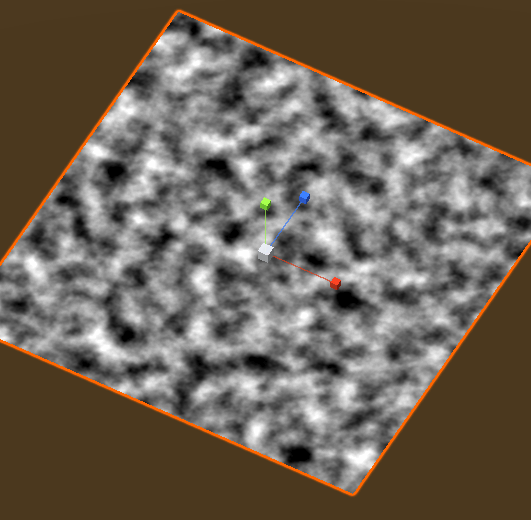## Tiling and UV space noise in 3d

For the example how to translate those concepts into 3d, I’m going to use voronoi noise. We start deleting the functions and properties that we used for perlin noise and don’t need anymore. That’s the roughness and persistance properties and the easing, perlin and layered noise functions. Then we add the copy in the code for voronoi noise from the tutorial about voronoi noise.

Then we need to expand our shader to handle 3d vectors in a few places. First we add a new property called the “height”. We then use it as the `Z` factor of the value we pass to the voronoi function. Because we expect the uv space to go from 0 to 1, I’ll also limit the height to be between 0 and 1. Then we change the modulo function to accept and return 3d vectors, the operators work the same way on 2d vectors as on 3d vectors, so we don’t have to change anything except the types.

``````Shader "Tutorial/029_material_baking/repeating_3d_voronoi" {
Properties {
_Height ("Z coordinate (height)", Range(0, 1)) = 0
_CellAmount ("Cell Amount", Range(1, 32)) = 2
_Period ("Repeat every X cells", Vector) = (4, 4, 4, 0)
}
Tags{ "RenderType"="Opaque" "Queue"="Geometry"}

Pass{
CGPROGRAM

//include useful shader functions
#include "UnityCG.cginc"

//define vertex and fragment shader
#pragma vertex vert
#pragma fragment frag

#pragma target 3.0

#include "Random.cginc"

#define OCTAVES 4

float _CellAmount;
float3 _Period;
float _Height;

//the object data that's put into the vertex shader
struct appdata{
float4 vertex : POSITION;
float2 uv : TEXCOORD0;
};

//the data that's used to generate fragments and can be read by the fragment shader
struct v2f{
float4 position : SV_POSITION;
float2 uv : TEXCOORD0;
};

float3 modulo(float3 divident, float3 divisor){
float3 positiveDivident = divident % divisor + divisor;
return positiveDivident % divisor;
}

//voronoi noise function from voronoi tutorial

//the vertex function
v2f vert(appdata v){
v2f o;
//convert the vertex positions from object space to clip space so they can be rendered
o.position = UnityObjectToClipPos(v.vertex);
o.uv = v.uv;
return o;
}

float4 frag (v2f i) : SV_TARGET{
float3 value = float3(i.uv, _Height) * _CellAmount;
//get noise and adjust it to be ~0-1 range
float noise = voronoiNoise(value).z;

return noise;
}
ENDCG
}
}
FallBack "Standard"
}
``````

Then we also change the voronoi function to respect the tiling. Because we not only use the cell for the generation of random numbers, but also for distance calculations in voronoi noise, we can’t simply make the cell itself tile. Instead we’ll make a separate variable which is a tiled version of the cell. Then we can use the cell itself for position calculations and the tiled cell for the random number generation. It’s important that we do this for both the cell generation in the first pass as well as the second pass where we calculate the distance from the edge.

``````float3 voronoiNoise(float3 value, float3 period){
float3 baseCell = floor(value);

//first pass to find the closest cell
float minDistToCell = 10;
float3 toClosestCell;
float3 closestCell;
[unroll]
for(int x1=-1; x1<=1; x1++){
[unroll]
for(int y1=-1; y1<=1; y1++){
[unroll]
for(int z1=-1; z1<=1; z1++){
float3 cell = baseCell + float3(x1, y1, z1);
float3 tiledCell = modulo(cell, period);
float3 cellPosition = cell + rand3dTo3d(tiledCell);
float3 toCell = cellPosition - value;
float distToCell = length(toCell);
if(distToCell < minDistToCell){
minDistToCell = distToCell;
closestCell = cell;
toClosestCell = toCell;
}
}
}
}

//second pass to find the distance to the closest edge
float minEdgeDistance = 10;
[unroll]
for(int x2=-1; x2<=1; x2++){
[unroll]
for(int y2=-1; y2<=1; y2++){
[unroll]
for(int z2=-1; z2<=1; z2++){
float3 cell = baseCell + float3(x2, y2, z2);
float3 tiledCell = modulo(cell, period);
float3 cellPosition = cell + rand3dTo3d(tiledCell);
float3 toCell = cellPosition - value;

float3 diffToClosestCell = abs(closestCell - cell);
bool isClosestCell = diffToClosestCell.x + diffToClosestCell.y + diffToClosestCell.z < 0.1;
if(!isClosestCell){
float3 toCenter = (toClosestCell + toCell) * 0.5;
float3 cellDifference = normalize(toCell - toClosestCell);
float edgeDistance = dot(toCenter, cellDifference);
minEdgeDistance = min(minEdgeDistance, edgeDistance);
}
}
}
}

float random = rand3dTo1d(closestCell);
return float3(minDistToCell, random, minEdgeDistance);
}
``````
``````float4 frag (v2f i) : SV_TARGET{
float3 value = float3(i.uv, _Height) * _CellAmount;
//get noise and adjust it to be ~0-1 range
float noise = voronoiNoise(value, _Period).z;

return noise;
}
``````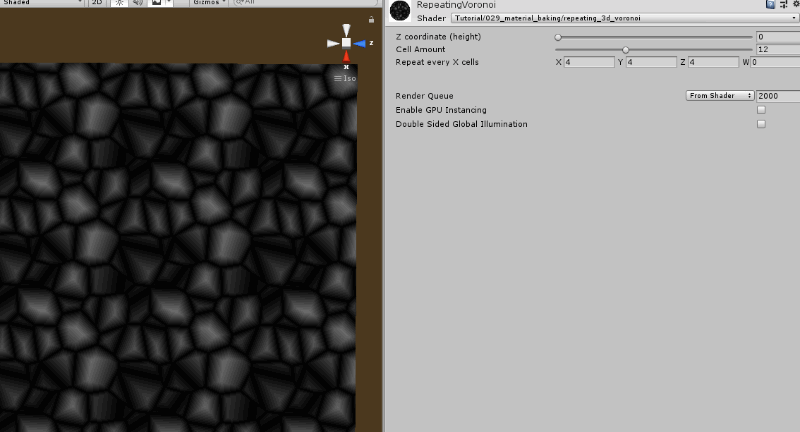## Source

### Tiling 2d layered perlin

``````Shader "Tutorial/029_material_baking/layered_repeating_perlin" {
Properties {
_CellAmount ("Cell Amount", Range(1, 32)) = 2
_Period ("Repeat every X cells", Vector) = (4, 4, 0, 0)
[IntRange]_Roughness ("Roughness", Range(1, 8)) = 3
_Persistance ("Persistance", Range(0, 1)) = 0.4
}
Tags{ "RenderType"="Opaque" "Queue"="Geometry"}

Pass{
CGPROGRAM

//include useful shader functions
#include "UnityCG.cginc"

//define vertex and fragment shader
#pragma vertex vert
#pragma fragment frag

#pragma target 3.0

#include "Random.cginc"

#define OCTAVES 4

float _CellAmount;
float _Roughness;
float _Persistance;
float2 _Period;

//the object data that's put into the vertex shader
struct appdata{
float4 vertex : POSITION;
float2 uv : TEXCOORD0;
};

//the data that's used to generate fragments and can be read by the fragment shader
struct v2f{
float4 position : SV_POSITION;
float2 uv : TEXCOORD0;
};

float easeIn(float interpolator){
return interpolator * interpolator;
}

float easeOut(float interpolator){
return 1 - easeIn(1 - interpolator);
}

float easeInOut(float interpolator){
float easeInValue = easeIn(interpolator);
float easeOutValue = easeOut(interpolator);
return lerp(easeInValue, easeOutValue, interpolator);
}

float2 modulo(float2 divident, float2 divisor){
float2 positiveDivident = divident % divisor + divisor;
return positiveDivident % divisor;
}

float perlinNoise(float2 value, float2 period){
float2 cellsMimimum = floor(value);
float2 cellsMaximum = ceil(value);

cellsMimimum = modulo(cellsMimimum, period);
cellsMaximum = modulo(cellsMaximum, period);

//generate random directions
float2 lowerLeftDirection = rand2dTo2d(float2(cellsMimimum.x, cellsMimimum.y)) * 2 - 1;
float2 lowerRightDirection = rand2dTo2d(float2(cellsMaximum.x, cellsMimimum.y)) * 2 - 1;
float2 upperLeftDirection = rand2dTo2d(float2(cellsMimimum.x, cellsMaximum.y)) * 2 - 1;
float2 upperRightDirection = rand2dTo2d(float2(cellsMaximum.x, cellsMaximum.y)) * 2 - 1;

float2 fraction = frac(value);

//get values of cells based on fraction and cell directions
float lowerLeftFunctionValue = dot(lowerLeftDirection, fraction - float2(0, 0));
float lowerRightFunctionValue = dot(lowerRightDirection, fraction - float2(1, 0));
float upperLeftFunctionValue = dot(upperLeftDirection, fraction - float2(0, 1));
float upperRightFunctionValue = dot(upperRightDirection, fraction - float2(1, 1));

float interpolatorX = easeInOut(fraction.x);
float interpolatorY = easeInOut(fraction.y);

//interpolate between values
float lowerCells = lerp(lowerLeftFunctionValue, lowerRightFunctionValue, interpolatorX);
float upperCells = lerp(upperLeftFunctionValue, upperRightFunctionValue, interpolatorX);

float noise = lerp(lowerCells, upperCells, interpolatorY);
return noise;
}

float sampleLayeredNoise(float2 value){
float noise = 0;
float frequency = 1;
float factor = 1;

[unroll]
for(int i=0; i<OCTAVES; i++){
noise = noise + perlinNoise(value * frequency + i * 0.72354, _Period * frequency) * factor;
factor *= _Persistance;
frequency *= _Roughness;
}

return noise;
}

v2f vert(appdata v){
v2f o;
//convert the vertex positions from object space to clip space so they can be rendered
o.position = UnityObjectToClipPos(v.vertex);
o.uv = v.uv;
return o;
}

float4 frag (v2f i) : SV_TARGET{
float2 value = i.uv * _CellAmount;
//get noise and adjust it to be ~0-1 range
float noise = sampleLayeredNoise(value) + 0.5;

return noise;
}
ENDCG
}
}
FallBack "Standard"
}
``````

### Tiling 3d voronoi

``````Shader "Tutorial/029_material_baking/repeating_3d_voronoi" {
Properties {
_Height ("Z coordinate (height)", Range(0, 1)) = 0
_CellAmount ("Cell Amount", Range(1, 32)) = 2
_Period ("Repeat every X cells", Vector) = (4, 4, 4, 0)
}
Tags{ "RenderType"="Opaque" "Queue"="Geometry"}

Pass{
CGPROGRAM

//include useful shader functions
#include "UnityCG.cginc"

//define vertex and fragment shader
#pragma vertex vert
#pragma fragment frag

#pragma target 3.0

#include "Random.cginc"

#define OCTAVES 4

float _CellAmount;
float3 _Period;
float _Height;

//the object data that's put into the vertex shader
struct appdata{
float4 vertex : POSITION;
float2 uv : TEXCOORD0;
};

//the data that's used to generate fragments and can be read by the fragment shader
struct v2f{
float4 position : SV_POSITION;
float2 uv : TEXCOORD0;
};

float3 modulo(float3 divident, float3 divisor){
float3 positiveDivident = divident % divisor + divisor;
return positiveDivident % divisor;
}

float3 voronoiNoise(float3 value, float3 period){
float3 baseCell = floor(value);

//first pass to find the closest cell
float minDistToCell = 10;
float3 toClosestCell;
float3 closestCell;
[unroll]
for(int x1=-1; x1<=1; x1++){
[unroll]
for(int y1=-1; y1<=1; y1++){
[unroll]
for(int z1=-1; z1<=1; z1++){
float3 cell = baseCell + float3(x1, y1, z1);
float3 tiledCell = modulo(cell, period);
float3 cellPosition = cell + rand3dTo3d(tiledCell);
float3 toCell = cellPosition - value;
float distToCell = length(toCell);
if(distToCell < minDistToCell){
minDistToCell = distToCell;
closestCell = cell;
toClosestCell = toCell;
}
}
}
}

//second pass to find the distance to the closest edge
float minEdgeDistance = 10;
[unroll]
for(int x2=-1; x2<=1; x2++){
[unroll]
for(int y2=-1; y2<=1; y2++){
[unroll]
for(int z2=-1; z2<=1; z2++){
float3 cell = baseCell + float3(x2, y2, z2);
float3 tiledCell = modulo(cell, period);
float3 cellPosition = cell + rand3dTo3d(tiledCell);
float3 toCell = cellPosition - value;

float3 diffToClosestCell = abs(closestCell - cell);
bool isClosestCell = diffToClosestCell.x + diffToClosestCell.y + diffToClosestCell.z < 0.1;
if(!isClosestCell){
float3 toCenter = (toClosestCell + toCell) * 0.5;
float3 cellDifference = normalize(toCell - toClosestCell);
float edgeDistance = dot(toCenter, cellDifference);
minEdgeDistance = min(minEdgeDistance, edgeDistance);
}
}
}
}

float random = rand3dTo1d(closestCell);
return float3(minDistToCell, random, minEdgeDistance);
}

v2f vert(appdata v){
v2f o;
//convert the vertex positions from object space to clip space so they can be rendered
o.position = UnityObjectToClipPos(v.vertex);
o.uv = v.uv;
return o;
}

float4 frag (v2f i) : SV_TARGET{
float3 value = float3(i.uv, _Height) * _CellAmount;
//get noise and adjust it to be ~0-1 range
float noise = voronoiNoise(value, _Period).z;

return noise;
}
ENDCG
}
}
FallBack "Standard"
}
``````

I hope I was able to explain how to make noise tile and that this knowledge will serve you well when you need it.

You can also find me on twitter at [@totallyRonja](https://www.twitter.com/totallyRonja). If you liked my tutorial and want to support me you can do that on Patreon (patreon.com/RonjaTutorials) or Ko-Fi (ko-fi.com/RonjaTutorials).